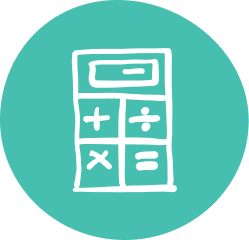### Quality Grade 7 Math Tutoring & Help

Oxford Learning®’s 7th Grade math tutoring program is specifically designed to help students develop improved comprehension.

Your child’s Grade 7 math tutor not only teaches math tips and techniques, but also how to think actively and approach math with more confidence–a skill that means learning success both in and out of the classroom.

Oxford Learning® offers more than quality Grade 7 math help: our cognitive approach helps your child learn how to learn, a skill that transfers from class to class. Our 7th Grade math tutors help make math make sense–so that students go from math frustration to math success.

### Grade 7 Math Tutoring Subjects

##### Number Sense/Numeration
• Representing and ordering decimals
• Fractions and integers
• Representing squares and square roots
• Dividing whole number by simple fractions and decimals,
• Adding and subtracting simple fractions and integers
• Multiplying and dividing decimal numbers to thousandths by 1 digit whole numbers
• Applying order of operations in expressions
• Solving problems involving whole number percents and unit rates
##### Measurement
• Converting between metric units
• Developing the area relationship for a trapezoid and prisms
##### Geometry & Spatial Sense
• Constructing parallel, perpendicular and intersecting lines
• Sorting and classifying triangles and quadrilaterals by geometric properties
• Constructing angle and perpendicular bisectors
• Relationships among congruent shapes
• Relating enlarging and reducing to similar shapes
• Comparing similar and congruent shapes
• Performing and describing dilatations
• Tiling a plane
• Plotting points in all four quadrants
##### Algebra & Patterning
• Representing linear growing patterns
• Representing patterns algebraically
• Modelling real life relationships involving constant rates graphically and algebraically
• Translating phrases using algebraic expressions
• Finding the term in a pattern algebraically
• Solving linear equations using concrete materials or inspections and guess and check
##### Data Management & Probability
• Collecting and organizing categorical,discrete and continuous data,
• Displaying data in relative frequency tables and circle graphs
• Identifying bias in data
• Relating changes in data to changes in central tendency
• Making inferences based on data
• Real world applications of probability
• Determining the theoretical probability of two independent events